# Calculating Discount Rate

This post will guide you how to calculate discount rate based on the discounted price and the original price in Excel. How do I generate percentage discount rate with a formula in Excel. How to calculate discount price in Excel.

## Calculating Discount Rate

Assuming that you have a list of data in range A1:B4 that contain original price of product and the current sales price (discounted price), and you want to get percentage discount rate. And how to achieve it. You just need to do the following steps:

#1 type the following formula in a blank cell (C2), then press Enter key in your keyboard.

`=(B2-A2)/ABS(A2)`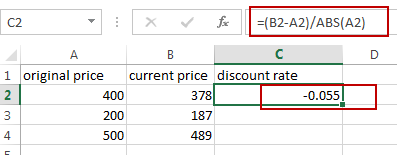#2 drag the AutoFill handle from the Cell C2 to C4 to apply the above formula.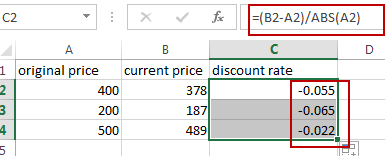#3 select all discount rate cells C2:C4, and then right click on it, select Format Cells, and the Format Cells dialog will open.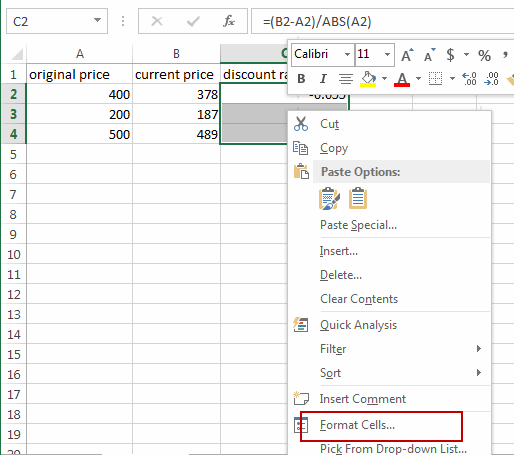#4 click Number tab, select Percentage in the category list box. And type 2 in the decimal places box, and then click OK button.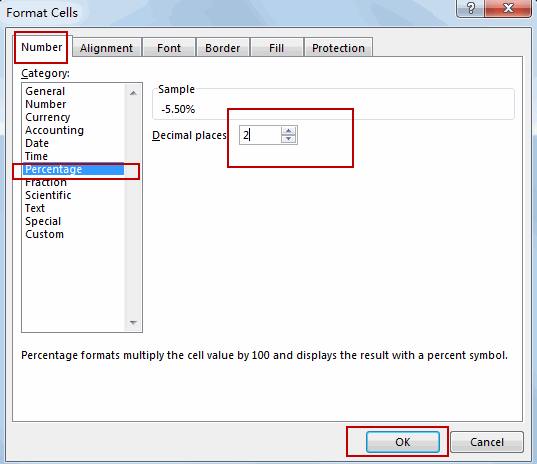#5 you will see that the discount percentage rate have been generated.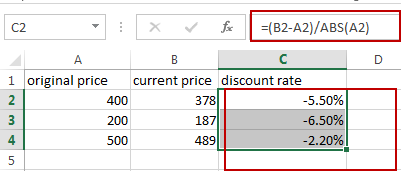Assuming that you have original prices and the discount rate in your data, and you want to calculate the current sales price or discounted price, you just need to use the following formula.

`=A2-(B2*A2)`

Type this formula in a blank cell and then press Enter key.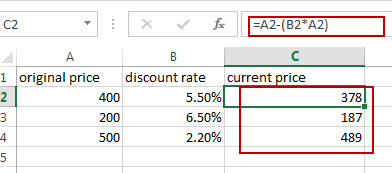### Related Functions

• Excel ABS Function
The Excel ABS function returns the absolute value of a number.The ABS function is a build-in function in Microsoft Excel and it is categorized as a Math and Trigonometry Function.The syntax of the ABS function is as below:=ABS (number)…